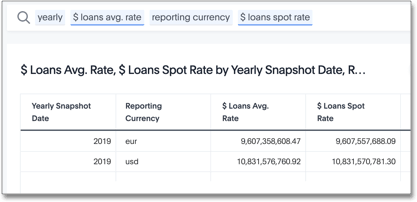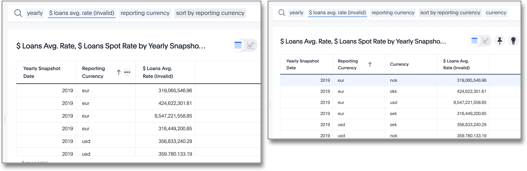# Reaggregation Scenario 2: Average rates of exchange

The Average rate of exchange calculates for the selected period. These average rates provide a mechanism to hedge the value of loans against price fluctuations in the selected period. We apply the average rate after the aggregation.

The pseudo-logic that governs the value of loans is `sum(loans) * average(rate)`.

The data model has two tables: a primary fact table, and a dimension table for `rates`.

• The `loans` column is from the primary fact table.

• The `rate` column is from the `rates` table.

These tables are at different levels of aggregation:

• The primary fact table uses a lower level of aggregation, on `product`, `department`, or `customer`.

• The `rates` dimension table use a higher level of aggregation, on `daily`, `transaction currency`, or `reporting currency`.

The two tables are joined through a relationship join on `date` and `transaction currency`.

To simplify the scenario, we only use a single `reporting currency`. The join ensures that a single rate value returns each day for each transaction currency.

## Valid solution

A valid query that meets our objective may look something like this:

```sum(group_aggregate (sum(loans)*average (rate),
query_groups () + {transaction_currency},
query_filters () ))```

The following search and resulting response returns the dollar value for each year, for each target reporting currency. Note that the dataset contains both euro (€) and US dollars (\$). The `\$ Loans Avg. Rate` calculates the average rate of exchange for the entire period. The `\$ Loans Spot Rate` applies the rate of exchange on the day of the transaction.## Resolution

1. The `sum(loans)` function aggregates to these attributes:

• `{transaction_currency}` and `query_groups()`

Add additional search columns to this aggregation. Here, this at the level of `reporting currency` and `year`.

• `query_filters( )`

Applies any filters entered in the search. Here, there are no filters.

2. Similarly, the `average(rate)` function aggregates to these attributes:

• `{transaction_currency}` and `query_groups()`

Add additional search columns to this aggregation. Here, this at the level of `reporting currency` and `year`.

• `query_filters( )`

Applies any filters entered in the search. Here, there are no filters.

3. For each row in this virtual table, the exchange rate applies to the sum of loans: `sum(loans) * average(rate)`.

4. The outer `sum()` function reaggregates the final output as a single row for each yearly reporting currency value.

 The default aggregation setting does not reaggregate the result set.

## Non-Aggregated Result

We include the following result to provide contrast to an example where ThoughtSpot does not reaggregate the result set. Reaggregation requires the aggregate function, `sum`, to precede the `group_aggregate` function.

In the following scenario, the formula assumes that the default aggregation applies. Here, the result returns 1 row for each `transaction currency`.

```group_aggregate (sum(loans )*average (rate ),
query_groups() + {transaction_currency},
query_filters())```